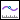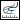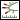Point—Evaluates a normal curvature vector at a datum point or a specified point on a curve or edge. Displays the curvature, normal, tangent, and radius at the selected point on the curve or edge. You can also specify a coordinate system.Radius—Evaluates and displays the minimum radius for a curve or edge at the selected point. The radius is equal to 1/curvature.Curvature—Evaluates and displays the curvature of curves or edges. Mathematically, the curvature is equal to 1/radius.Offset—Evaluates and displays an offset for a selected set of curves or edges. You must also select a reference plane to display the offset.Deviation—Evaluates and displays the deviation from a curve or edge to a datum point, curve, or datum point array.